So… The moment we've all been waiting for!

The `CALCULATE` function is a special function in that it is the only one which can alter filter contexts.

This means we can update, add and remove filter context, ignoring that which has already been applied.

Let's see what this means in practice... If we use this expression to evaluate the number of children in London: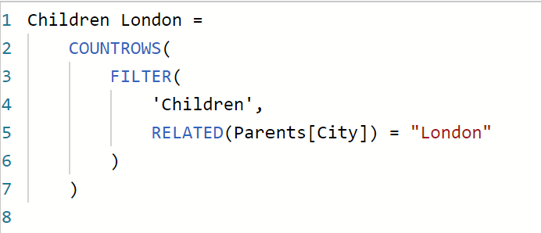Here we evaluate expressions from inner to outer. The inner `FILTER` function is returning the children table but filtered so that only the rows whose parents are in London are returned. This is done using the `RELATED` function to access the correct rows in the parents table. If we display this on an aggregated visual, then it will show up as follows: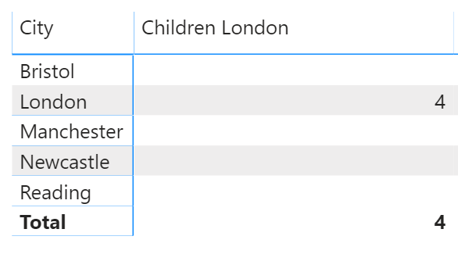Each of these aggregated rows is applying its own filter context. So, for example, for the Bristol row the input dataset will be the children dataset with the filter context of `RELATED(Parents[City]) = "Bristol"` applied. We are then filtering this dataset using `RELATED(Parents[City]) = "London"` in our expression. Therefore, the resulting dataset it empty and the cell is blank.

If we instead used the following expression: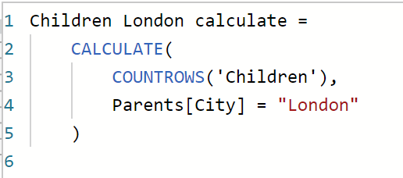Then any outer filter context is ignored and replaced by the one supplied to `CALCULATE`. Therefore, the context applied by each row is therefore ignored and the table will look like this: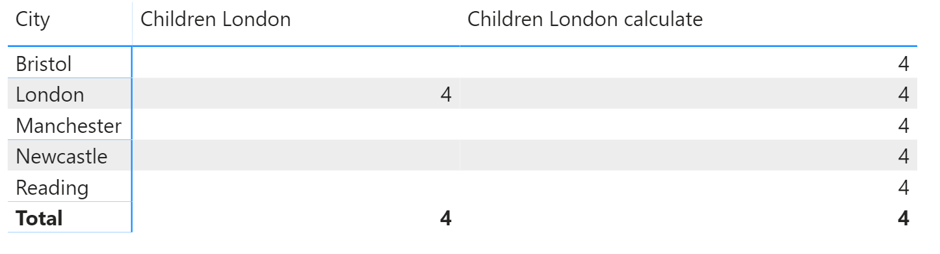In every row the filter context has been replaced by `Parents[City] = "London"` and the same value is displayed in each cell. Notice that here we don't need to use `RELATED`. This is because `CALCULATE` works on the whole model not on individual tables.

I think the functionality is easier to understand once we see that any filter expression applied is actually a table.

For example, when you write the above expression you are actually writing: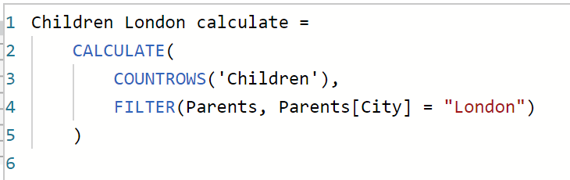Here we can see that instead of just "applying a new filter context", we are in fact handing the function a whole new table. The short-hand version is more easily readable but I think it's important to realise what's actually happening.

This is also important because it means that we can actually hand the function any table. We can use this to remove the filters from, for example, just one column. If we use the visuals to filter down to those born in the last 25 years and also living in London, we can see that we are left with 3 children: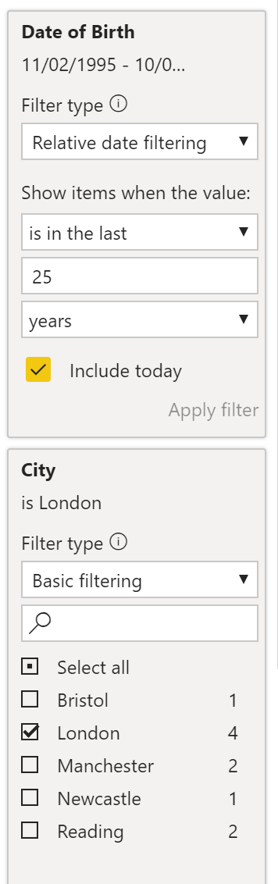But say we wanted to see the total number of children born in the past 25 years, irrespective of what location filters are applied. Then we could use the following to remove the filtering by city: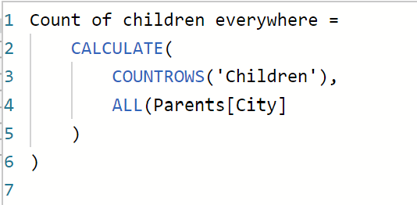We can see that this will ignore the regional filtering and produce a count of 11 children despite having the same filters applied to the page as a whole.In this way we can remove the filtering on individual columns. This means that we have a lot of control and flexibility over the data we display.

## Context transition

There is one final property of `CALCULATE` which we need to talk about. Context transition. When used inside a row context, `CALCULATE` transitions that row context into a corresponding filter context.

This can be seen using our original calculated column example. If we revert our model to a single table model, where the number of children is just a column in the parents table: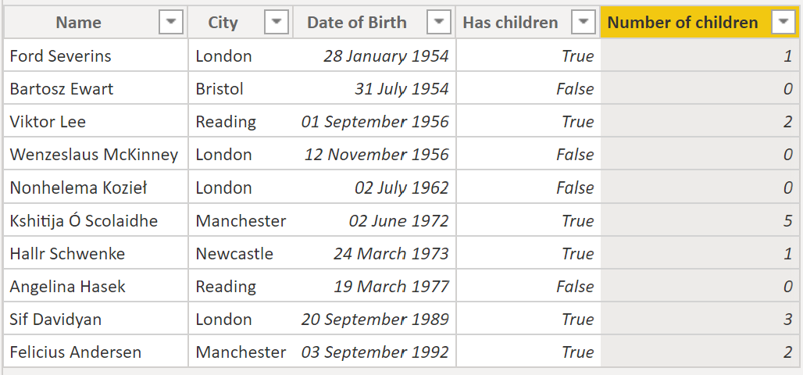Remember the first calculated column we made, which summed the total number of children:The same value here is displayed for each row because the `SUM` ignores the row context. However, if we wrap the expression in a `CALCULATE`: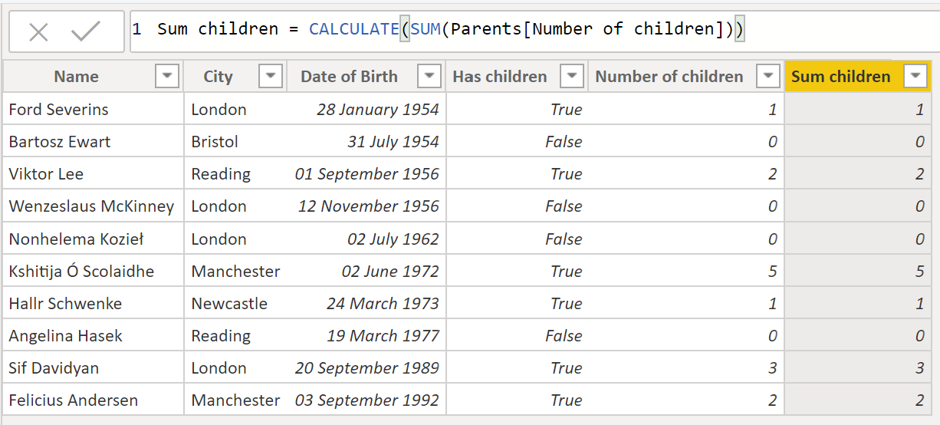Then we can see that the row context has now been taken into account when calculating the `SUM` for each row. This is because `CALCULATE` has transformed that row context into a corresponding filter context, which will then be seen by the `SUM`. So, for example, for the first row `CALCULATE` has translated the row context so that the `SUM` is acting over a dataset of just the one row, and therefore is evaluated as 1. The same is then true for each individual row.

This is important to remember when using calculate in combination with iterative functions, or you can end up with surprising results!

The final thing that we need to highlight is the effect of using measures. If we instead define a measure to calculate the sum (without using `CALCULATE`):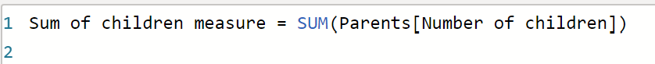And then use that measure to define a calculated column: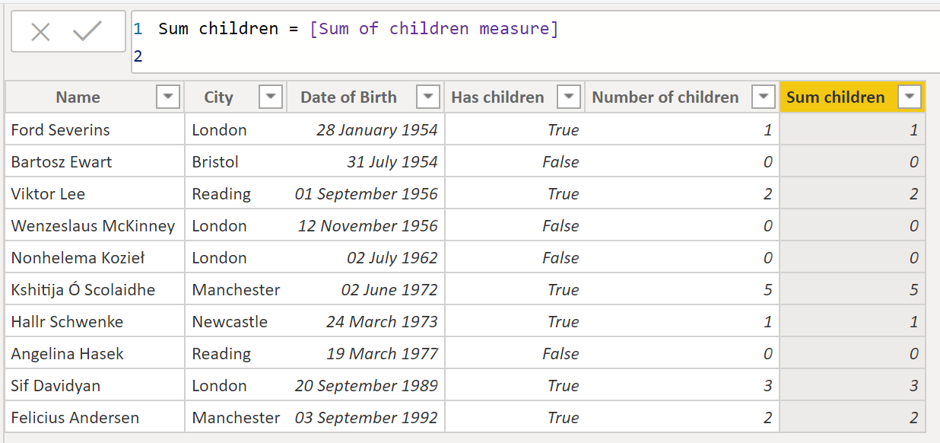Notice that the row context is still taken into account.

This is because when you use measures inside formulae, they are automatically wrapped in a `CALCULATE`. This is very important to remember when using measures in combination with iterative functions, otherwise you can end up with some unexpected results just by moving parts of the calculation out into a measure!

### Carmel Eve

#### Software Engineer I